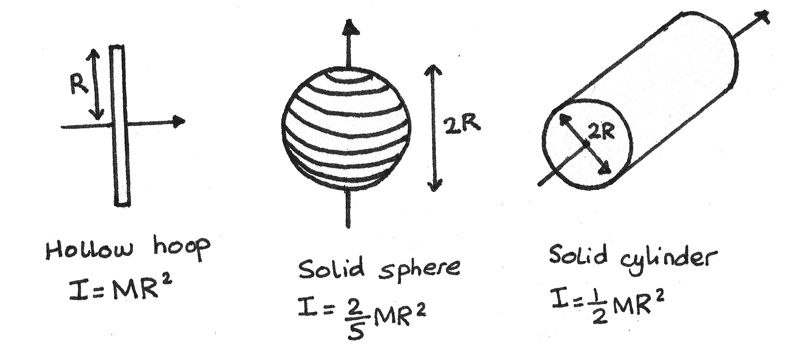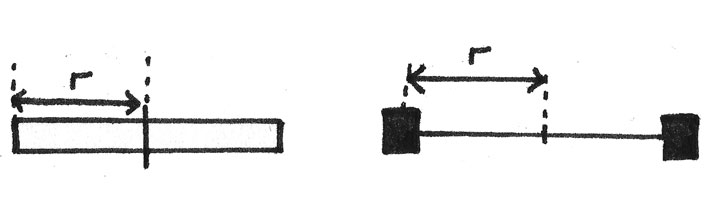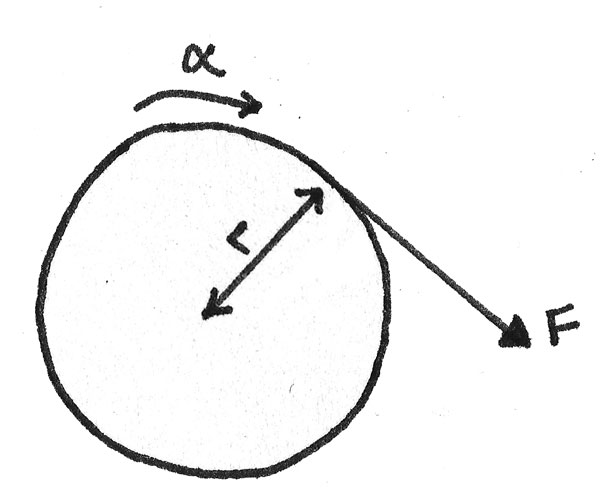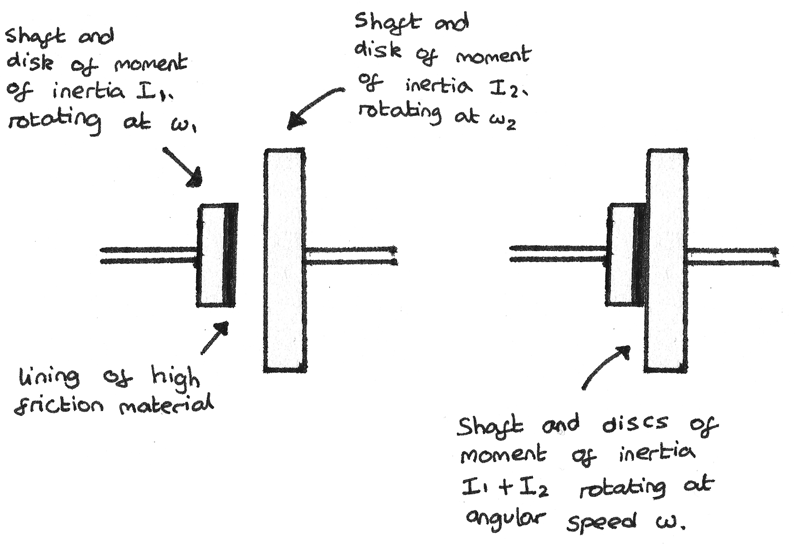# Rotational Dynamics

Rotational dynamics can be used to describe an object travelling in circular motion while linear dynamics describe an object travelling in a straight line. In rotational dynamics, angles and angular displacements are measured in radians instead of degrees.

### Angular Displacement

Angular displacement is the angle an object has rotated around a circle relative to a fixed axis. It can be calculated by:

$$\theta = \frac{S}{r}$$

Where $\theta$ is the angle in radians, $S$ is the arc length around the circle and $r$ is the radius of the circle.

### Angular Velocity

Angular velocity is the angular displacement travelled per second.

$$\omega = \frac{\Delta{\theta}}{\Delta{t}}$$

Where $\omega$ is the angular velocity ($rad~s^{-1}$), $\theta$ is the angular displacement ($rad$) and $t$ is the time ($s$). It can also be calculated using the time period $T$ of a rotation:
$$\omega = \frac{2\pi}{T}$$

An increase in the angular velocity results in an increase in the linear velocity:
$$v = r \omega$$
Where $v$ is the linear velocity ($ms^{-1}$), $r$ is the radius of the circle ($m$) and $\omega$ is the angular velocity ($rad~s^{-1}$).

### Angular Acceleration

Angular acceleration is the rate of change of angular velocity.

$$\alpha = \frac{\Delta{\omega}}{\Delta{t}}$$

Where $\alpha$ is the angular acceleration ($rad~s^{-2}$), $\omega$ is the angular velocity ($rad~s^{-1}$) and $t$ is the time ($s$). An increase in the angular acceleration results in an increase in the linear acceleration:
$$a = r\alpha$$
Where $a$ is the linear acceleration ($ms^{-2}$), $r$ is the radius of the circle ($m$) and $\alpha$ is the angular acceleration ($rad~s^{-2}$).

## Moment of Inertia

The dynamics of a rotating object depend on the distribution of mass relative to a particular axis of rotation. An object can be considered as being made up of an infinite number of point masses of mass $m_{i}$ each a distance $r_{i}$ away from a central axis.

The rotational kinetic energy of a single point mass will therefore be:
$$\frac{1}{2}m_{1}v_{1}^{2} = \frac{1}{2}m_{1}{(\omega r_{1})}^{2} = \frac{1}{2}\omega^{2} m_{1}r_{1}^{2}$$

Adding together all the individual kinetic energies gives the total kinetic energy of the object:

$$E_{k} = \frac{1}{2}\omega^{2} m_{1}r_{1}^{2} + \frac{1}{2}\omega^{2} m_{2}r_{2}^{2} + \cdots + \frac{1}{2}\omega^{2} m_{i}r_{i}^{2}$$

As the angular velocity $\omega$ is equal for all of the point masses:
\eqalign{ E_{k} &= \frac{1}{2}\omega^{2}(m_{1}r_{1}^{2} + m_{2}r_{2}^{2} + \cdots + m_{i}r_{i}^{2}) \\ E_{k} &= \frac{1}{2}\omega^{2}(\Sigma m_{i}r_{i}^{2})}

The $\Sigma m_{i}r_{i}^{2}$ term is defined as the moment of inertia, which is a measure of the resistance of an object to an angular acceleration.

$$I = \Sigma m_{i}r_{i}^{2}$$

The units of $I$ are $kg~m^{2}$. Different shaped objects have different expressions for the moment of inertia.If two objects share a common axis of rotation, the sum of the moments of inertia gives the combined moment of inertia for the system. For two flywheels of moments of inertia $\frac{1}{2}M_{A}R_{A}^{2}$ and $\frac{1}{2}M_{B}R_{B}^{2}$, the total moment of inertia of the system is:
$$I = \frac{1}{2}M_{A}R_{A}^{2} + \frac{1}{2}M_{B}R_{B}^{2}$$

## Rotational Kinetic Energy

As shown above, the rotational kinetic energy of an object rotating around a given axis is:

\eqalign{ E_{k} &= \frac{1}{2}\omega^{2}(\Sigma m_{i}r_{i}^{2}) \\ E_{k} &= \frac{1}{2}I\omega^{2} }

Where $E_{k}$ is the rotational kinetic energy ($J$), $I$ is the moment of inertia ($kgm^{2}$) and $\omega$ is the angular velocity ($rad~s^{-1}$). It is important to ensure that the rotational velocity is converted from $rev~min^{-1}$ to $rad~s^{-1}$.

### Flywheels

Flywheels are used to store rotational kinetic energy. Some uses of flywheels are shown below:

• Flywheel batteries can be used for as an emergency electrical backup supply. In the event of a power failure, a rotating flywheel acts as a generator.
• Flywheels can be used to even out fluctuations in rotational speed during a cycle of an internal combustion energy. Flywheels maintain a constant rotational velocity between the power strokes of the pistons.
• Flywheels can be used for regenerative braking in vehicles where the energy absorbed during braking is reused to accelerate the car.

#### Factors Affecting Energy Store

The maximum energy store of a flywheel is given by the maximum rotational kinetic energy of the flywheel:

$$E_{k} = \frac{1}{2}I\omega^{2} = \frac{1}{2}\omega^{2}(\Sigma m_{i}r_{i}^{2})$$

This equation shows that the maximum rotational kinetic energy depends on the angular velocity ($\omega$) and the moment of inertia ($I$). The rotational kinetic energy can be increased by:

• Rotating the flywheel faster which increases the angular velocity $\omega$. The maximum possible angular velocity depends on the breaking stress of the material.
• Increasing the moment of inertia $I$ of the flywheel. As the formula for the moment of inertia is $I = \Sigma m_{i}r_{i}^{2}$, this can be done by concentrating the mass $m$ at the greatest value of $r$.Flywheels that use spokes to concentrate most of its mass as a ring on the outside will have a greater moment of inertia than a solid disc of equal mass.

## Equations of Rotational Motion

There are four equations of rotational motion that are similar to the equations of linear motion. The quantities used are shown below:

• $\omega_{1}$ is the angular velocity at the start ($rad~s^{-1}$).
• $\omega_{2}$ is the angular velocity at the end ($rad~s^{-1}$).
• $\theta$ is the change in angular displacement ($rad$).
• $\alpha$ is the constant angular acceleration ($rad~s^{-2}$).
• $t$ is the time ($s$).

The equations of rotational motion are shown below:

\eqalign{ \omega_{2} &= \omega_{1} + \alpha t \\ \theta &= \omega_{1} t + \frac{1}{2}\alpha t^{2} \\ \omega_{2}^{2} &= \omega_{1}^{2} + 2\alpha \theta \\ \theta &= \frac{1}{2}(\omega_{1} + \omega_{2})t }

### Torque

The torque is the product of the force and perpendicular distance of the line of action of the force from a fixed axis.$$T = Fs$$

Where $T$ is the torque ($Nm$), $F$ is the applied force ($N$) and $s$ is the perpendicular distance ($m$). For most rotating systems, this is the radius of the circle. Using Newton’s second law, the torque can be expressed in terms of the moment of inertia of the object:

$$T = I\alpha$$

Where $T$ is the torque ($Nm$), $I$ is the moment of inertia ($kgm^{2}$) and $\alpha$ is the angular acceleration ($rad~s^{-2}$).

## Angular Momentum

The angular momentum of a rotating object is a vector quantity acting parallel to the angular velocity defined as:
$$L = I\omega$$
Where $L$ is the angular momentum ($Nms$), $I$ is the moment of inertia ($kgm^{2}$) and $\omega$ is the angular velocity ($rad~s^{-1}$).

Likewise to linear momentum, the conservation of angular momentum states that when no external torque acts on a closed system, the total angular momentum remains constant.

This means that if the moment of inertia $I$ increases by concentrating an object’s mass at a greater distance from the central axis, the angular velocity of the object must decrease to keep the angular momentum constant. An example of this is a dancer extending their arms while rotating in order to slow themselves down.

A clutch is another example of the conservation of angular momentum in action:Before the engagement, the total angular momentum is:
$$L_{\text{before}} = I_{1}\omega_{1} + I_{2}\omega_{2}$$
After the engagement, the total angular momentum is:
$$L_{\text{after}} = (I_{1} + I_{2})\omega_{2}$$
Due to the conservation of angular momentum:
\eqalign{ L_{\text{before}} &= L_{\text{after}} \\ I_{1}\omega_{1} + I_{2}\omega_{2} &= (I_{1} + I_{2})\omega}

### Angular Impulse

An angular impulse, $\Delta L$ is a change in angular momentum, $L$.

$$\Delta L = I\omega_{2} - I\omega_{1}$$
Where $\Delta L$ is the change in momentum ($Nms$), $I$ is the moment of inertia ($kgm^{2}$), $\omega_{1}$ is the angular velocity ($rad~s^{-1}$) before the change in momentum and $\omega_{2}$ is the angular velocity ($rad~s^{-1}$) after the change in momentum.

When the moment of inertia is constant, an angular impulse can be related to torque by the expression:

$$T = I\alpha = I\frac{\Delta \omega}{\Delta t} = \frac{\Delta L}{\Delta t} \\ \\ \Delta L = T\Delta t$$

This equation shows that the torque acting acting over a time interval is equal to the angular impulse.

### Work Done

In rotational dynamics, the work done is the product of the force applied and the angular displacement. For a rotating system, the work done can be defined as:

$$W = T \theta$$

Where $W$ is the work done ($J$), $T$ is the torque applied ($Nm$), and $\theta$ is the angle the object has rotated through. Work may be done to overcome resistive forces or to increase the angular velocity of the rotating object.

### Power

As power is the rate of doing work, it can be defined as:
$$P = \frac{\Delta W}{\Delta t} = T \frac{\Delta \theta}{\Delta t}$$
Where $\frac{\Delta \theta}{\Delta t}$ is the rate of change of angular displacement. The power can therefore be defined as:
$$P = T\omega$$
Where $P$ is the power ($W$), $T$ is applied torque ($Nm$) and $\omega$ is the angular velocity ($rad~s^{-1}$).